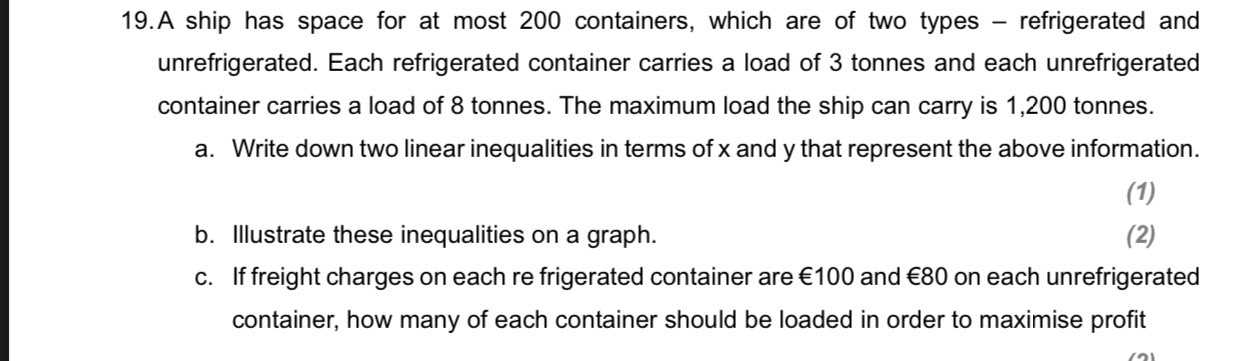### ¿Todavía tienes preguntas de matemáticas?

Pregunte a nuestros tutores expertos
Algebra
Pregunta19. A ship has space for at most $$200$$ containers, which are of two types - refrigerated and unrefrigerated. Each refrigerated container carries a load of $$3$$ tonnes and each unrefrigerated container carries a load of $$8$$ tonnes. The maximum load the ship can carry is $$1,200$$ tonnes. a. Write down two linear inequalities in terms of $$x$$ and y that represent the above information. b. Illustrate these inequalities on a graph. c. If freight charges on each re frigerated container are $$€ 100$$ and $$€ 80$$ on each unrefrigerated (1) container, how many of each container should be loaded in order to maximise profit